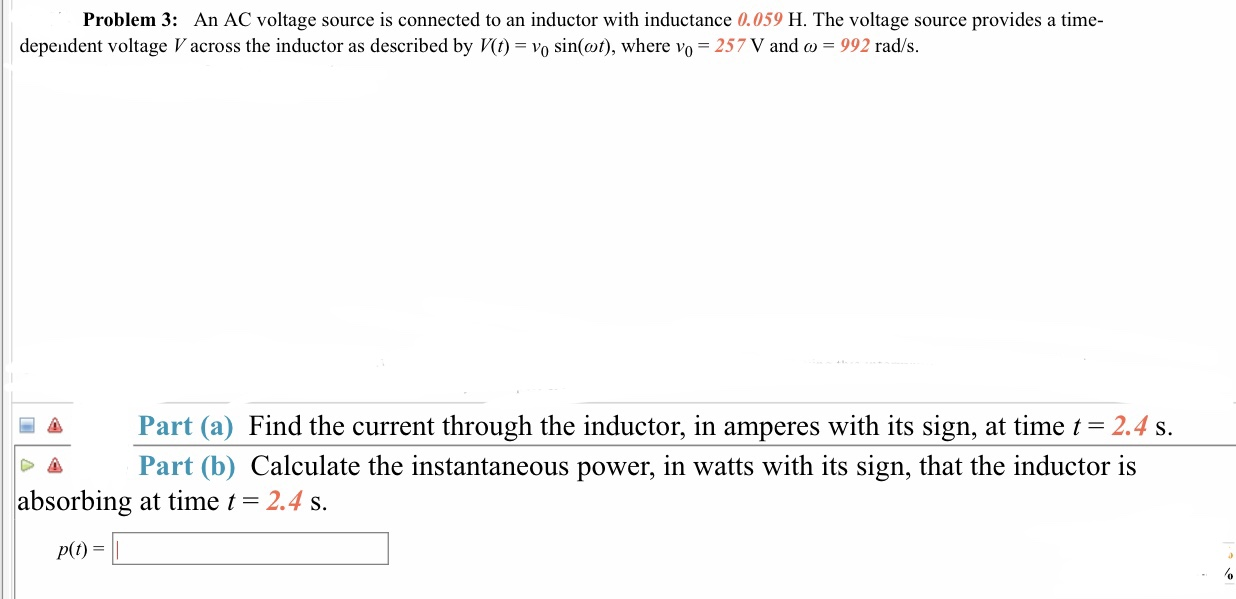# Problem 3: An AC voltage source is connected to an inductor with inductance 0.059 H. The voltage source provides a time- dependent voltage V across the inductor as described by Vt) - Vo sin(ot), where vo- 257 V and co - 992 rad/s. Part (a) Find the current through the inductor, in amperes with its sign, at time t 2.4 s Part (b) Calculate the instantaneous power, in watts with its sign, that the inductor is absorbing at time t = 2.4 s. P(t)

Questionhelp_outlineImage TranscriptioncloseProblem 3: An AC voltage source is connected to an inductor with inductance 0.059 H. The voltage source provides a time- dependent voltage V across the inductor as described by Vt) - Vo sin(ot), where vo- 257 V and co - 992 rad/s. Part (a) Find the current through the inductor, in amperes with its sign, at time t 2.4 s Part (b) Calculate the instantaneous power, in watts with its sign, that the inductor is absorbing at time t = 2.4 s. P(t) fullscreen

### Want to see this answer and more?

Experts are waiting 24/7 to provide step-by-step solutions in as fast as 30 minutes!*

*Response times vary by subject and question complexity. Median response time is 34 minutes and may be longer for new subjects.
Tagged in
SciencePhysics

### Alternating Current